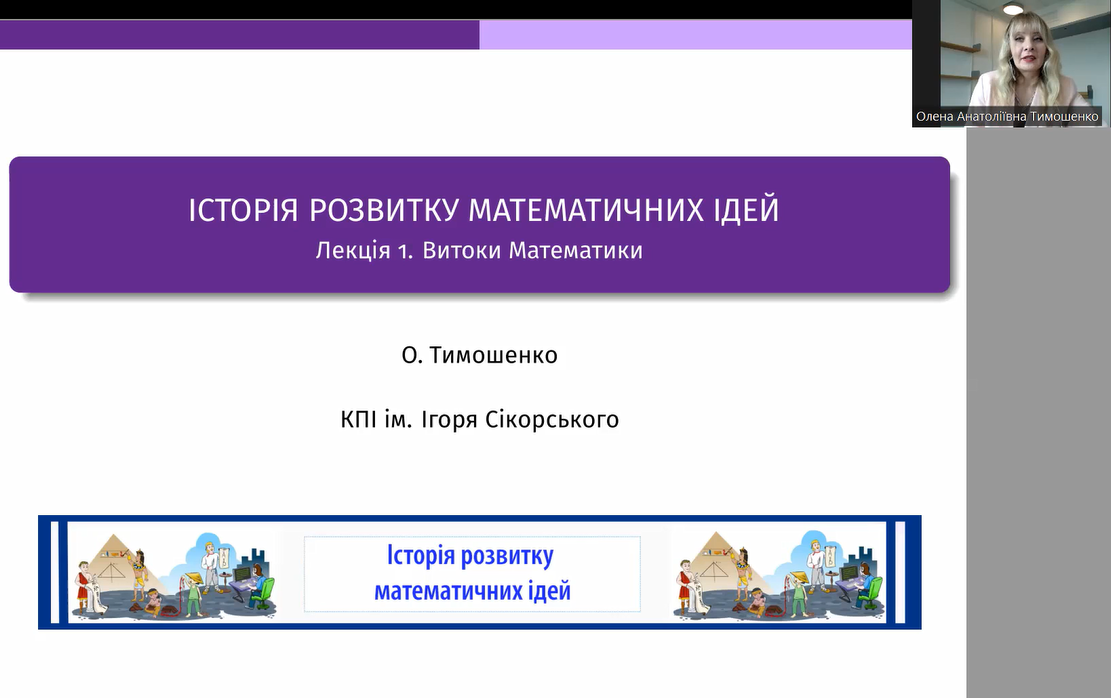## Courses

### Natural Science > MathematicsCourse
History of the development of mathematical ideas
Lecturer
Tymoshenko, Olena
University
Igor Sikorsky Kyiv Polytechnic Institute
Period
Fall 2022
Language
Ukranian

### Syllabus

Methods of mathematical research are used in a wide variety of fields of knowledge. In this course you will see firsthand many of the results that have made what mathematics is today and meet the mathematicians that created them. The course provides an overview of some of mathematical ideas during the history, say classical problems of ancient Greek mathematics lead to involved mathematical theories including transcendent numbers or Galois theory; chinese double regula falsi method transforms to modern Newton-Raphson method of finding roots of nonlinear equations; babylonian ideas of solving quadratic equations turns out be utilized in modern algebra; arabic tables of approximate values of trigonometric functions are useful nowadays in deep procedures of numerical mathematics and so on. All of these is explained in the corresponding lectures of the course. The course will survey major mathematical developments beginning with ancient Greeks and tracing the development through Hindu-Indian mathematics, Arabic mathematics, and European mathematics. This course helps students to understand better the modern mathematical ideas by discovering their roots in the history and to develop their critical thinking and research skills.